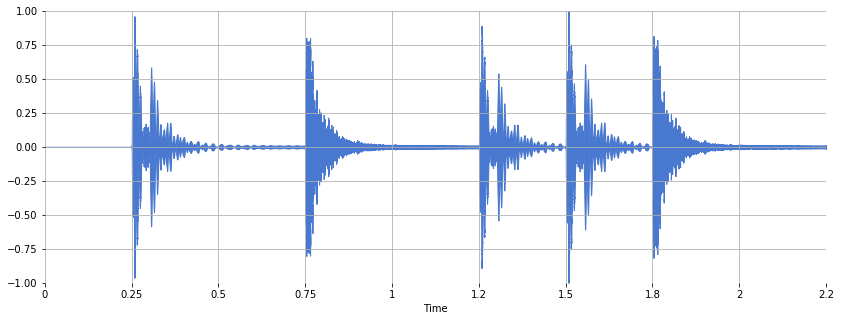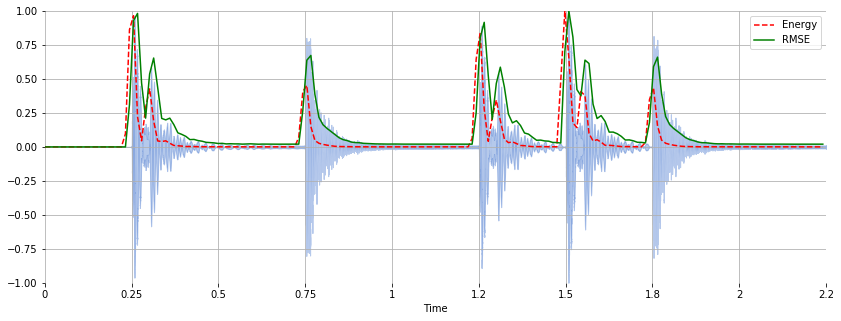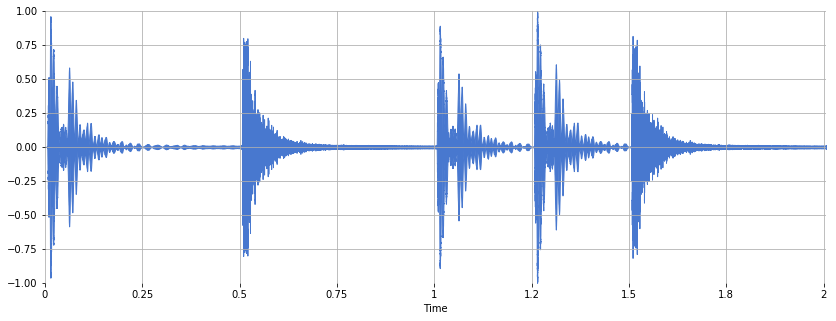# Energy and RMSE¶

The energy (Wikipedia; FMP, p. 66) of a signal corresponds to the total magntiude of the signal. For audio signals, that roughly corresponds to how loud the signal is. The energy in a signal is defined as

$$\sum_n \left| x(n) \right|^2$$

The root-mean-square energy (RMSE) in a signal is defined as

$$\sqrt{ \frac{1}{N} \sum_n \left| x(n) \right|^2 }$$

In :
x, sr = librosa.load('audio/simple_loop.wav')

In :
sr

Out:
22050
In :
x.shape

Out:
(49613,)
In :
librosa.get_duration(x, sr)

Out:
2.2500226757369615

Listen to the signal:

Out:

Plot the signal:

Out:
<matplotlib.collections.PolyCollection at 0x10cd21cc0>Compute the short-time energy using a list comprehension:

In :
hop_length = 256
frame_length = 512

In :
energy = numpy.array([
sum(abs(x[i:i+frame_length]**2))
for i in range(0, len(x), hop_length)
])

In :
energy.shape

Out:
(194,)

Compute the RMSE using librosa.feature.rmse:

In :
rmse = librosa.feature.rmse(x, frame_length=frame_length, hop_length=hop_length, center=True)

In :
rmse.shape

Out:
(1, 194)
In :
rmse = rmse


Plot both the energy and RMSE along with the waveform:

In :
frames = range(len(energy))
t = librosa.frames_to_time(frames, sr=sr, hop_length=hop_length)

Out:
<matplotlib.legend.Legend at 0x10cd54cc0>## Questions¶

Write a function, strip, that removes leading silence from a signal. Make sure it works for a variety of signals recorded in different environments and with different signal-to-noise ratios (SNR).

In :
def strip(x, frame_length, hop_length):

# Compute RMSE.
rmse = librosa.feature.rmse(x, frame_length=frame_length, hop_length=hop_length, center=True)

# Identify the first frame index where RMSE exceeds a threshold.
thresh = 0.01
frame_index = 0
while rmse[frame_index] < thresh:
frame_index += 1

# Convert units of frames to samples.
start_sample_index = librosa.frames_to_samples(frame_index, hop_length=hop_length)

# Return the trimmed signal.
return x[start_sample_index:]


Let's see if it works.

In :
y = strip(x, frame_length, hop_length)

Out:
Out:
<matplotlib.collections.PolyCollection at 0x10ce20128>It worked!